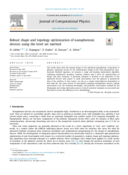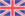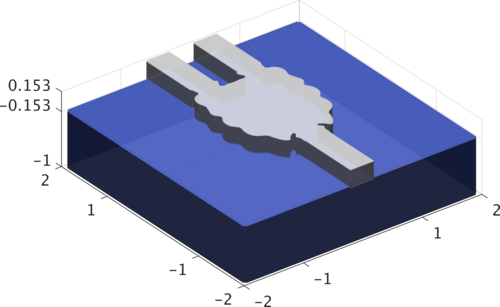# Shape optimization for nanophotonic

Ph.D thesis (started in october 2016)

## Nanophotonic components

Nano-photonic devices are the key component in the fabrication of many integrated photonic circuit, used for example in the telecommunications field.

These devices are composed of many structured layers of high optical index material (silicon, germanium ...) surrounded by others (silica, silicon nitride, air ...) of lower index. This high contrast between the material allows the confinement of the light (the principle is similar to the one used in optical fibers). Depending on the materials distribution inside the device, it is possible to treat the light in order to, for example, redirect, modulate, sort in function of the wavelength (demultiplexer) or divide the incident power between multiple outputs.

Classical nanophotonic devices are composed of several geometric shapes with characteristic sizes varying from a few dozen to several hundreds of micrometers. Currents lithography-etching processes even allow the inclusion of patterns with sizes of about ten nanometers. At this scale, the approximation of geometrical optic are no longer sufficiently precise to describe lightwave propagation. Thus, it is necessary to see the light as an electromagnetic wave whose behavior is mathematically described by the Maxwell equations. $$\nabla \times \nabla \times \mathbf{E} - k^2 n^2 \mathbf{E} = 0$$

## Shape optimization problem

Geometric and topologic shape optimization methods have been applied to the photonic field for about ten to twenty years, but practical realization of devices with micrometric dimensions have been achieved only recently.

In my Ph.D. I am mainly interested in geometric shape optimization methods which have the advantage of being able to easily obtain properties such as the normal or the local curvature at the edges. This type of optimization is based on the Hadamard shape derivative concept. In a nutshell, this allows us to determine a vector field $$\mathbf{\theta}$$ such that by moving the shape of the device according to this field will lead to a new shape (that is to say a new device) which is more efficient.

All the details of this method are available in our paper:
• #### « Robust shape and topology optimization of nanophotonic devices using the level set method »Status: published in Journal of Computational Physics, Volume 395, 710–746 (2019) [ article, preprint]

Authors: Nicolas Lebbe, Charles Dapogny, Edouard Oudet, Karim Hassan and Alain Glière

Keywords: Shape optimization · Level-set method · Nanophotonics · Robustness · Maxwell equations

Abstract:... This paper deals with shape optimization of nanophotonic devices subject to some environmental or fabrication uncertainties. In order to obtain robust components according to such uncertainties, we devised a method based on a multi-objectives algorithm together with the classical level-set shape optimization. A particular attention was paid to the case of manufacturing variations coming from the etching and lithography steps that the integrated optics community considers to be of major importance in the design of nanophotonic devices. Our article presents numerous numerical examples validating on the one hand our multi-objectives algorithm and on the other hand the robust optimization procedure.

An example of a result obtained with this method is given in Figure 1 and many other results can be viewed in my thesis.Fig 1: Optimization of a power splitter, power loss = 0.01 W = -0.03 dB